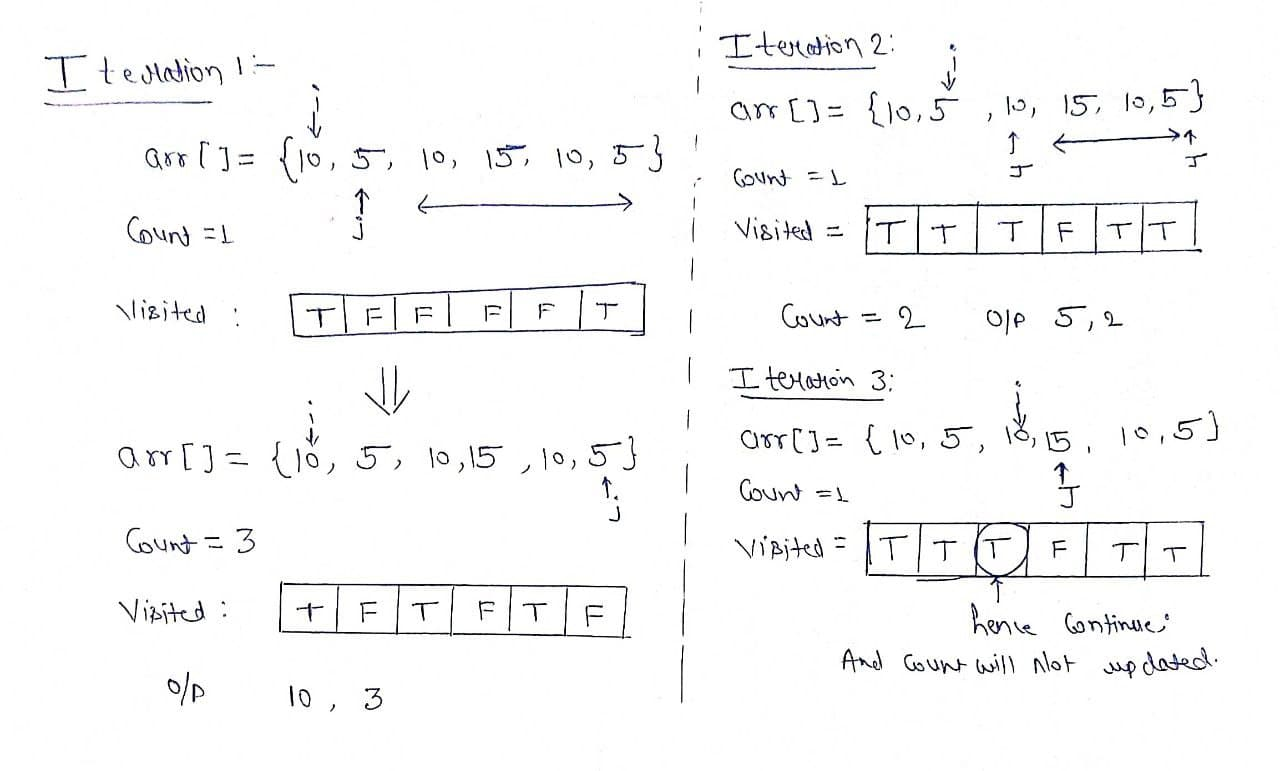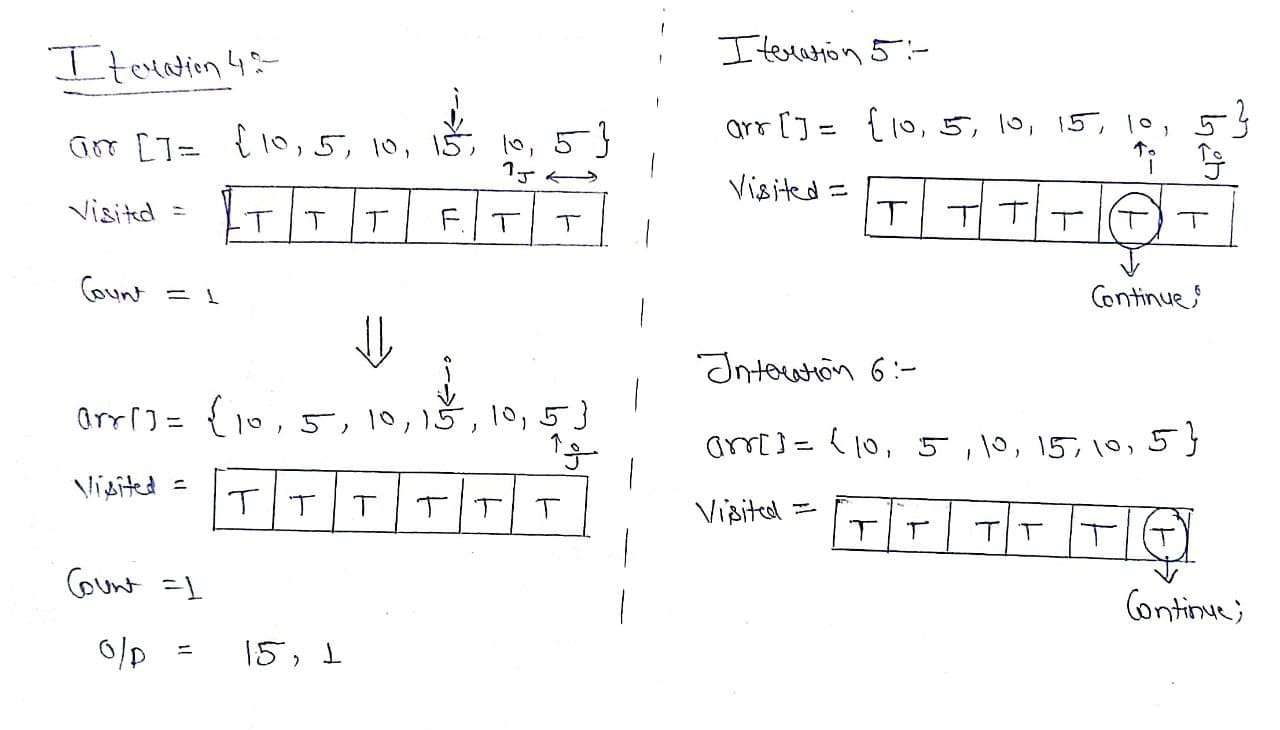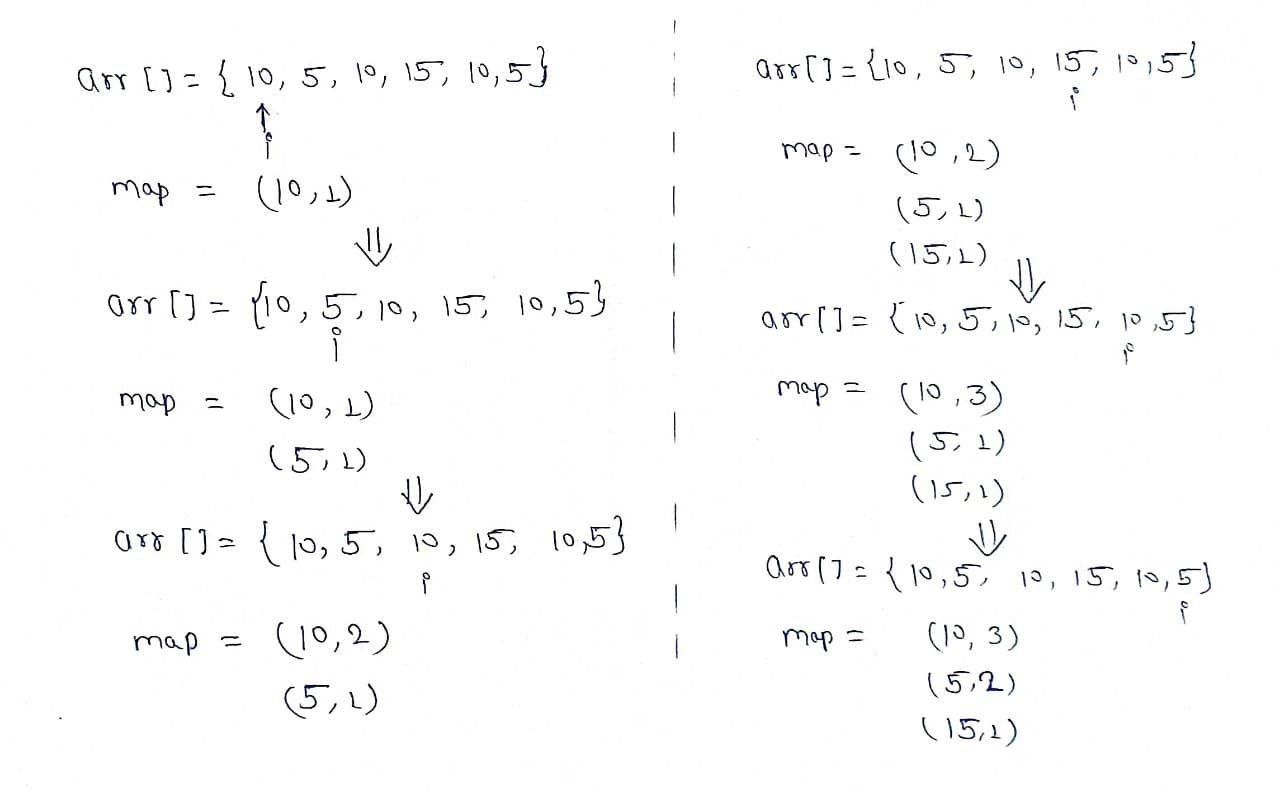# Count frequency of each element in the array

Problem statement: Given an array, we have found the number of occurrences of each element in the array.

Examples:

```Example 1:
Input: arr[] = {10,5,10,15,10,5};
Output: 10  3
5  2
15  1
Explanation: 10 occurs 3 times in the array
5 occurs 2 times in the array
15 occurs 1 time in the array

Example2:
Input: arr[] = {2,2,3,4,4,2};
Output: 2  3
3  1
4  2
Explanation: 2 occurs 3 times in the array
3 occurs 1 time in the array
4 occurs 2 time in the array
```

### Solution

Disclaimer: Don’t jump directly to the solution, try it out yourself first.

Solution 1: Use of two loops

Intuition: We can simply use two loops, in which the first loop points to one element and the second loop finds that element in the remaining array. We will keep track of the occurrence of that same element by maintaining a count variable. We also have to maintain a visited array so that it will keep track of the duplicate elements that we already count.

Approach:

• Make a visited array of type boolean.
• Use the first loop to point to an element of the array.
• Initialize the variable count to 1.
• Make that index true in the visited array.
• Run second loop, if we find the element then mark the visited index true and increase the count.
• If the visited index is already true then skip the other steps.Code:

## C++ Code

``````#include <bits/stdc++.h>
using namespace std;

void countFreq(int arr[], int n)
{
vector<bool> visited(n, false);

for (int i = 0; i < n; i++) {

// Skip this element if already processed
if (visited[i] == true)
continue;

// Count frequency
int count = 1;
for (int j = i + 1; j < n; j++) {
if (arr[i] == arr[j]) {
visited[j] = true;
count++;
}
}
cout << arr[i] << " " << count << endl;
}
}

int main()
{
int arr[] = {10,5,10,15,10,5};
int n = sizeof(arr) / sizeof(arr);
countFreq(arr, n);
return 0;
}
``````

Output:

10 3
5 2
15 1

Time Complexity: O(N*N)

Space Complexity:  O(N)

## Java Code

``````import java.util.*;

public class tuf {

public static void main(String args[]){

int arr[] ={10,5,10,15,10,5};
int n = arr.length;
countFreq(arr, n);
}
public static void countFreq(int arr[], int n)
{
boolean visited[] = new boolean[n];

for (int i = 0; i < n; i++) {

// Skip this element if already processed
if (visited[i] == true)
continue;

// Count frequency
int count = 1;
for (int j = i + 1; j < n; j++) {
if (arr[i] == arr[j]) {
visited[j] = true;
count++;
}
}
System.out.println(arr[i] + " " + count);
}
}
}
``````

Output:

10 3
5 2
15 1

Time Complexity: O(N*N)

Space Complexity:  O(N)

Solution 2: Using Map

Intuition: We can use a map of value and frequency pair, in which we can easily update the frequency of an element if it is already present in the map, if it is not present in the map then insert it in the map with frequency as 1. After completing all the iterations, print the value frequency pair.

Approach:

• Take a unordered_map/HashMap of <Integer , Integer> pair.
• Use a for loop to iterate the array.
• If the element is not present in the map then insert it with frequency 1, otherwise increase the existing frequency by 1.
• Print the value frequency pair.Code:

## C++ Code

``````#include <bits/stdc++.h>
using namespace std;

void Frequency(int arr[], int n)
{
unordered_map<int, int> map;

for (int i = 0; i < n; i++)
map[arr[i]]++;

// Traverse through map and print frequencies
for (auto x : map)
cout << x.first << " " << x.second << endl;
}

int main()
{
int arr[] = {10,5,10,15,10,5};
int n = sizeof(arr) / sizeof(arr);
Frequency(arr, n);
return 0;
}
``````

Output:

15 1
10 3
5 2

Time Complexity: O(N)

Space Complexity: O(n)

## Java Code

``````import java.util.*;

public class tuf {

public static void main(String args[]){

int arr[] = {10,5,10,15,10,5};
int n = arr.length;
Frequency(arr, n);
}
static void Frequency(int arr[], int n)
{
Map<Integer, Integer> map = new HashMap<>();

for (int i = 0; i < n; i++)
{
if (map.containsKey(arr[i]))
{
map.put(arr[i], map.get(arr[i]) + 1);
}
else
{
map.put(arr[i], 1);
}
}
// Traverse through map and print frequencies
for (Map.Entry<Integer,Integer> entry : map.entrySet())
{
System.out.println(entry.getKey() + " " + entry.getValue());
}
}
}
``````

Output:

5 2
10 3
15 1

Time Complexity: O(N)

Space Complexity: O(n)

Special thanks to Prashant Sahu for contributing to this article on takeUforward. If you also wish to share your knowledge with the takeUforward fam, please check out this article